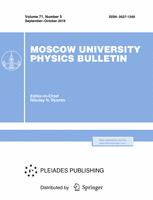Faculty of Physics
M.V.Lomonosov Moscow State University

# The modeling of magnetoplasma structures in the solar wind

## E. V. Maiewski$^1$, R. A. Kislov$^{2,3}$, H. V. Malova$^{2,4}$, O. V. Khabarova$^3$, V. Yu. Popov$^{2,5,6}$, A. A. Petrukovich$^2$

### Memoirs of the Faculty of Physics 2018. N 5.

• Article
Annotation

Axisymmetric MHD model of the solar wind is constructed, which allows us to study the spatial distribution of the magnetic field and plasma characteristics at radial distances from 20 to 400 radii of the Sun in a wide range of heliolatitudes. Self-consistent solutions for the magnetic field, plasma and current densities in the solar wind depending on the phase of the solar cycle are obtained. It is shown that during the dominance of the dipole magnetic component in the solar wind, a heliospheric current sheet (HCS) is formed, being the part of a system of longitudinal and transverse currents symmetric in the Northern and Southern hemispheres. As the relative contribution of a quadrupole component to the total magnetic field of HTS increases, the last one becomes to be cone-shaped; at high latitudes of the opposite hemisphere, a second conical HTS appears, its opening angle increases. The model describes a smooth transition from the fast solar wind at high heliolatitudes to the slow solar wind at low heliolatitudes and the steepened profiles of main solar wind characteristics with the increased radial distance from the Sun

Approved: 2018 December 10
PACS:
52.65.-y Plasma simulation
96.50.Ci Solar wind plasma; sources of solar wind
96.60.-j Solar physics
02.70.-c Computational techniques; simulations
Rissian citation:
Е. В. Маевский, Р. А. Кислов, Х. В. Малова, О. В. Хабарова, В. Ю. Попов, А. А. Петрукович.
Учен. зап. физ. фак-та Моск. ун-та. 2018. № 5. 1850307.
Authors
E. V. Maiewski$^1$, R. A. Kislov$^{2,3}$, H. V. Malova$^{2,4}$, O. V. Khabarova$^3$, V. Yu. Popov$^{2,5,6}$, A. A. Petrukovich$^2$
$^1$Department of data analysis, decision-making and financial technologies, Financial University under the Government of Russian Federation.
$^2$Space Research Institute RAS
$^3$The Schmidt Institute of Physics of the Earth of the Russian Academy of Sciences
$^4$M.V.Lomonosov Moscow State University, Skobeltsyn Institute of Nuclear Physics (SINP MSU)
$^5$Faculty of Physics, M.V. Lomonosov Moscow State University.
$^6$National Research University Higher School of Economics”### Moscow University Physics Bulletin### Science News of the Faculty of Physics, Lomonosov Moscow State University

This new information publication, which is intended to convey to the staff, students and graduate students, faculty colleagues and partners of the main achievements of scientists and scientific information on the events in the life of university physicists.